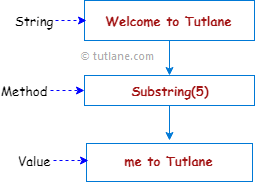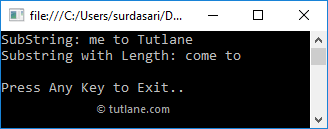# Visual Basic String Substring Method

In visual basic, the Substring method is useful to get a substring from the given string. The substring starts from the specified character position and continues to the end of the string.

In case, if we want to get the particular length of substring, we can specify the length of the substring to return from the given string.

Following is the pictorial representation of using the Substring() method to get a substring from the given string in a visual basic programming language.If you observe the above diagram, we are getting a substring starting from position 5 using the Substring method.

## Visual Basic Substring Method Syntax

Following is the syntax of defining a Substring method to get a substring from the given string in a visual basic programming language.

Public Function Substring(ByVal startIndex As Integer) As String

Public Function Substring(ByVal startIndex As Integer, ByVal length As Integer) As String

If you observe the syntax, the Substring method will return a substring starting from the specified position.

## Visual Basic Substring Method Example

Following is the example of using the Substring() method to get a substring starting from the given string in a visual basic programming language.

Module Module1

Sub Main()

Dim msg As String = "Welcome to Tutlane"

Console.WriteLine("SubString: {0}", msg.Substring(5))

Console.WriteLine("Substring with Length: {0}", msg.Substring(3, 7))

Console.WriteLine("Press Enter Key to Exit..")

End Sub

End Module

If you observe the above example, we used a Substring() method to get a substring starting from the position 5 till the end of the defined string and another substring starting from the position 3 and the length of substring should be 7 characters.

When we execute the above visual basic program, we will get the result as shown below.If you observe the above result, the Substring() method has returned a required substring with or without specified length based on our requirements.

This is how we can use the Substring() method to get required substrings from the given string starting from the defined position in a visual basic programming language.